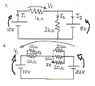# Basic Circuits Question

• Engineering
• suspenc3
If it is positive, then you found the current is flowing in the correct direction.Assuming that current flows in one direction will not give you a wrong answer, it will give you either a positive or negative answer. If it is negative, it simply means that the current is flowing in the opposite direction than you assumed, but you didn't get the "wrong answer". If it is positive, then you found the current is flowing in the correct direction.f

#### suspenc3

http://img213.imageshack.us/img213/6926/ccts3lg1.th.jpg [Broken]

I understand how to solve these...kinda, how do you know what way the voltages are flowing? I always thought that wou were just suppose to draw a direction and the equations will work themselves out (Or is this just for resistor voltages?)

How do you know what way the voltages from the batteries are flowing, so I can set up KVL equations?

Thanks.

#### Attachments

•ccts3.JPG
31.6 KB · Views: 341
Last edited by a moderator:
http://img213.imageshack.us/img213/6926/ccts3lg1.th.jpg [Broken]

I understand how to solve these...kinda, how do you know what way the voltages are flowing? I always thought that wou were just suppose to draw a direction and the equations will work themselves out (Or is this just for resistor voltages?)

How do you know what way the voltages from the batteries are flowing, so I can set up KVL equations?

Thanks.

Your image isn't showing up, but i'll try to answer your q's without. You assume a direction for the current, if the direction is not given. If they don't give you the polarity of the voltage source, then you just have to assume which side is + or -.

Last edited by a moderator:
I just attached it too, it might take a while to get approved though. I drew in my guesses, i think that the long line is (+) and the short line is (-)?

Also, I really don't understand thevenin equivalents, do you know of any good online tutorials or anything?All the book examples only have resistors in the circuits, but I have questions with a bunch of batteries, resistors and current sources.

Last edited:
Just like summing up forces and torques, you are supposed to arbitrarily guess a direction for each current and be consistent with it. If the math gives you a negative answer, then you know the current actually flows in the opposite direction

yeah, but doing that gives you the wrong answers, at least "assuming" the direction of battery voltages, don't you only "assume" resistor voltage directions? I think I figured out how to know what direction it flows in though (batteries).

Yea, you don't assume polarities for resistor voltages. What I meant about assuming polarities for voltages sources, is when the question asks you find the polarity of the voltage source. In your case, the polarities are given.

i think that the long line is (+) and the short line is (-)?

That's right. You need to learn what the standard symbols for drawing circuit diagrams mean, otherwise you will not make much progress! The main point of having standard symbols is to give the all the information clearly, and without needing words to explain the details every time.

yeah, but doing that gives you the wrong answers, at least "assuming" the direction of battery voltages, don't you only "assume" resistor voltage directions? I think I figured out how to know what direction it flows in though (batteries).

some sources will absorb power, and some will supply power. You can't always tell by looking at it which way the current is flowing, and thus you must assume a direction and solve from there. Assuming that current flows in one direction will not give you a wrong answer, it will give you either a positive or negative answer. If it is negative, it simply means that the current is flowing in the opposite direction than you assumed, but you didn't get the "wrong answer".